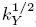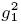## Articulo

•Citado por SciELO
•Accesos

•Similares en SciELO

## versión impresa ISSN 0035-001X

#### Resumen

DIAZ-CRUZ, J.L.  y  ROSADO, A.. Electroweak-Higgs unification in the two Higgs doublet model: masses and couplings of the neutral and charged Higgs bosons. Rev. mex. fis. [online]. 2007, vol.53, n.5, pp.396-406. ISSN 0035-001X.

We obtain the mass spectrum and the Higgs self-couplings of the two Higgs doublet model (THDM) in an alternative unification scenario where the parameters of the Higgs potential λi (i = 1, 2, 3, 4, 5) are determined by imposing their unification on the electroweak gauge couplings. An attractive feature of this scenario is the possibility of determining the Higgs boson masses by evolving the from the λi electroweak-Higgs unification scale MGH down to the electroweak scale. The unification condition for the gauge (1,2) and Higgs couplings is written as1 =2 =ƒ(λi), where1 =Y, and kY isthe normalization constant. Two variants for the unification condition are discussed: Scenario I is defined through the linear relation:1 =2 = kH(i) λi (MGH), while Scenario II assumes a quadratic relation== kH(i) λi (MGH). In Scenario I, by setting ad hoc -kH (5) =(4) =(3) = kH (2) = kH(1) = 1, taking tan β = 1 and using the standard normalization (kY = 5/3), we obtain the following spectrum for the Higgs boson masses:= 109.1 GeV,= 123.2 GeV,= 115.5 GeV, andH± = 80.3 GeV, with similar results for other normalizations such as kY = 3/2 and kY = 7/4.

Palabras llave : Electroweak-Higgs unification; Higgs boson masses and couplings; Two Higgs doublet model.

· resumen en Español     · texto en Inglés     · Inglés (pdf )Todo el contenido de esta revista, excepto dónde está identificado, está bajo una Licencia Creative Commons